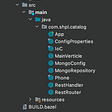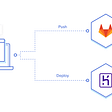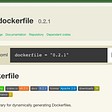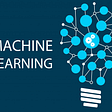# Fit Nonlinear Data with a Linear Model!

Fitting nonlinear data with a linear model is a technique called Polynomial Regression. The intuition is that the model will have a higher degree of freedom to fit the data.

First, we generate the data (note that y is a quadratic function of X):

`m = 100X = 9 * np.random.rand(m, 1) - 7y = X**2 + 3*X + 5 + np.random.randn(m, 1)`

The linear regression model (without Polynomial features):

`reg = LinearRegression()reg.fit(X, y)`

`poly= PolynomialFeatures(degree=2, include_bias=False)X_poly = poly.fit_transform(X)`

The first five samples from X:

`>>> X[:5]array([[-0.63502308]       [-6.87887923],       [-4.63090189],       [ 0.23522634],       [-5.11050991]])`

The first five samples from X_poly:

`>>> X_poly[:5]array([[-0.63502308,  0.40325431],       [-6.87887923, 47.31897949],       [-4.63090189, 21.4452523 ],       [ 0.23522634,  0.05533143],       [-5.11050991, 26.11731159]])`

The linear regression model (with Polynomial features):

`reg.fit(X_poly, y)`
`reg.intercept_, reg.coef_ #--> 4.84, 3.04, 1.01`

The models’ coefficients are almost identical to y.

This trick has many applications in machine learning (such as Support Machine Vectors). However, polynomial features can cause over-fitting. The solution is to use grid search to pick the optimal parameter for the polynomial feature function.

Bonus: The grid search implementation is in the link below:

https://github.com/booletic/medium/blob/main/poly.ipynb

--

--

--

## More from Mansoor Aldosari

https://github.com/booletic

Love podcasts or audiobooks? Learn on the go with our new app.

## Refactoring my app into modern Java with JSON + Rich Domain Model.## Shifting to Remote Dev. & QA Teams: Is It Temporary or Permanent?## Kollect x Satoshi Club AMA Recap from 27th of September## Pop!_OS Floating Window Exception## COBOL INSPECT Statement, COBOL string-manipulation guide.## How to Build an Effective Initial Deployment Pipeline## Transitioning Project OWL into Owl Integrations## Dynamically Generating Dockerfiles for K8s## Mansoor Aldosari

https://github.com/booletic

## Choosing the right graph## Using Machine learning Algorithm in Heart Failure Clinical Records Dataset## Normal Distribution and Normality Test## Introduction to Machine Learning